# Is that an affine transformation

## Geometric transformations

### 1 Introduction

There are many applications for geometric transformations, e.g. in the measurement of real objects using digital images. The CCD sensors used are very precise, so that precise measurements are possible.

In order to be able to convert the coordinates of the original into those of an image or model, a mapping rule is required that delivers the desired result. This requires a mathematical model that defines the geometric relationship (e.g. projection) between the original image and the image.

### Possible questions

• What are the image coordinates for a given mapping rule (e.g. central projection)?
• The archetype and image are known. What is the transformation? One application is the measurement of lenses.
• How can inaccuracies in the image, e.g. caused by the discrete pixel structure, be interpolated? Edges should not become "angular" and no invisible disturbances should arise.
• Is there a more efficient algorithm for the same problem?

### Geometric Transformation Requirements

In general, a geometric transformation should relate the pixel coordinates of the original image and the image. In many cases it will be a question of mapping the real world in a pixel image. Position, size, distance and relative arrangements (e.g. parallelism) play a role here. For example, if photographic material is available, it may be desirable to remove radial distortions caused by the lens system (fish-eye effect).

Often times, the next step is to zoom in or out. The question to be answered is how additional pixels are filled. Relative dimensions should also be retained, which has limits in the pixel area. What makes the accuracy of technical components possible should not be called into question by imprecise calculations.

After the original has been reproduced in a suitable form, e.g. with a stereo camera in the form of two two-dimensional pixel images, a three-dimensional image could be calculated from it.

### 2) Map forward and backward

Geometric transformations are mappings from one set of points to another.

A major problem arises from the fact that the grids of the original image and image generally do not match. There are two sub-problems:

• The pixels from the raster of the original image are normally not mapped exactly onto those of the image, but lie somewhere in between. If the pixels are understood as small squares, then several images of pixels touch the same pixel of the image grid. It is therefore necessary to interpolate in a suitable manner.
• The pre-image and the image have different numbers of pixels. A relation between a pixel of the original image and a pixel set of the image or vice versa is required; that is, a pixel must be assigned to several or vice versa. Here too, suitable interpolation should be used.

Let f be a mapping between two sets of pixels, P and P 'pixels with P archetype, P' image. Then there are two ways of doing this:

### a) P '= f (P)

We take the original image pixels and map them onto the image grid. In general, the image pixel P 'will lie somewhere between the grid positions. Now we could assign P 'to the closest pixel. Some pixels of the image grid might not be hit at all, others might be hit several times.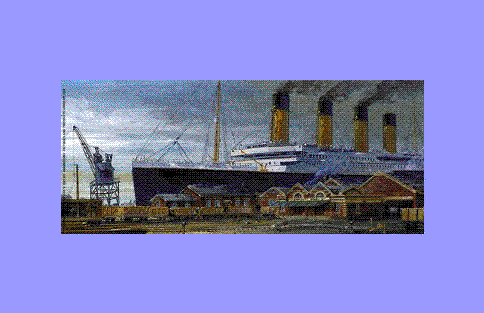The light pattern in the scaled image is created by the translucent background in places where no image of a pre-image pixel has been assigned to pixels of the image grid.

It would be better to distribute P 'over several pixels. This is possible if we think of each pixel as a square. A pixel in the image grid would then be averaged from all the image pixels covering it, with the percentage of coverage being taken as the weight. A relatively complex calculation would be necessary.

Both methods do not solve the problem of holes in the image.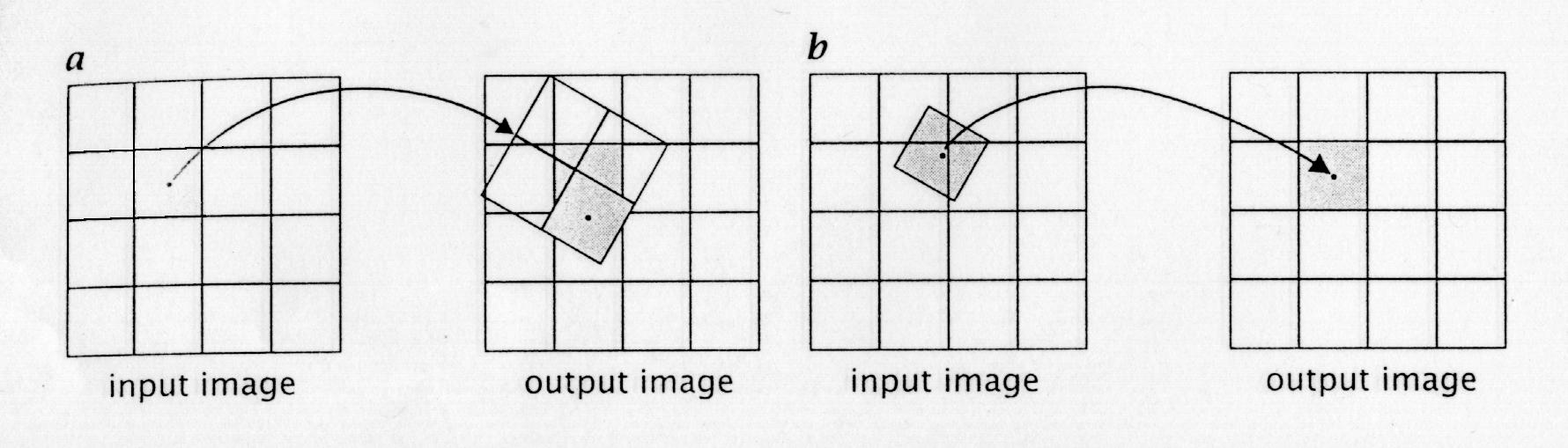a) P '= f (P) The image of the original image grid (drawn diagonally) does not coincide with the image grid. The four pixels could be used to calculate the value of the image pixel.
b) P = f -1 (P ') The original image of the pixel (drawn obliquely) touches several original image pixels.

### b) P = f -1 (P ')

A much better method is to start from the image grid and look for the location in the original image grid for each pixel P 'which is mapped onto P' by the mapping f. It will therefore determine the arithmetic original image for each image pixel. For this purpose, the reverse image f -1used. This way, no holes can appear in the picture. The interpolation problem is now in the original. Since the calculated points P = f -1 (P ') lie between the pixels of the original image grid, an algorithm must assign a meaningful value to them. This can be the value of the closest or an average of the neighboring pixel values, or it can be calculated from the surroundings of the original image pixel.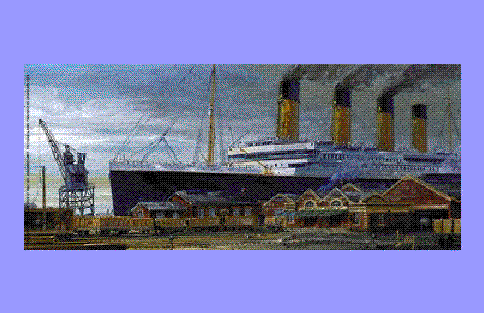Scaling using the inverse mapping method without interpolation.

The pixel value of the pixel closest to the arithmetic original image is used in each case.
Therefore, no holes appear in the image.

### 3) Linear and affine maps

If a point P is understood as a vector v, the linear images (with regard to vector addition and multiplication by a scalar) include rotation, scaling, stretching and shearing. The translation is not linear and belongs together with the linear to the affine transformations.

For a linear mapping f, applied to the points P and Q and the scalar k, the following applies:
• f (k P) = k f (P)
• f (P + Q) = f (P) + f (Q).

For an affine mapping g, a vector T and P, Q, k as above, the following applies:

• g (k P) = k g (P) + T
• g (P + Q) = g (P) + f (Q) + T.

Affine mappings have a number of useful properties, such as maintaining parallelism, and can be calculated using matrix calculations.

### 3.1) Determine image vectors

Linear mappings in vector spaces can be calculated by matrix multiplication; a vector is added for the translation. For points (x, y) in R² we get for an affine mapping:

v '= M * v + T ,

where v archetype, v 'image, M in R² a 2x2 matrix of the linear mapping, T translation vector,

or more precisely:These transformations can also be expressed with just one matrix multiplication if one goes to homogeneous coordinates.

A point P in R² can be represented by exactly one vector v = (x, y) with Cartesian coordinates. When using homogeneous coordinates, a coordinate is added. A point then corresponds to a whole array of vectors v = k * (x, y, 1). We use the representative (x, y, 1). In R³ the vectors have one more coordinate accordingly.

An affine mapping can be calculated as follows:

v '= A * v ,

where v archetype, v 'image, A in R² is a 3x3 matrix of affine mapping

or more precisely:a11, a12, a21, a22 determine the linear part of the transformation, tx and ty the translation.

In this way, the image can be calculated if the original image and transformation matrix are given.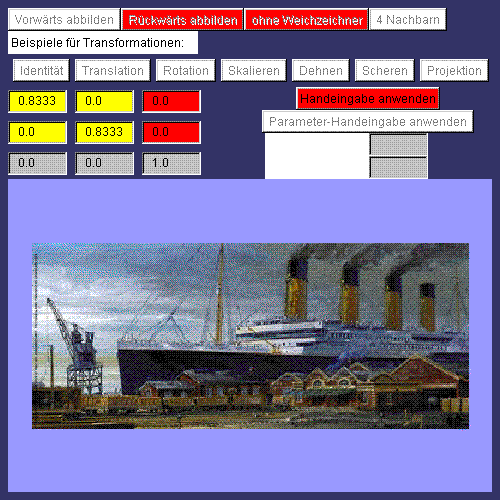At www.inf.fu-berlin.de/~vratisla/Bildverarbeitung/Transfrm/Transfrm.html, various functions mentioned in the text can be carried out in two-dimensional with this applet.

Functionality:

• The transformations translation, rotation, scaling, stretching, shear;
• All transformations using the normal and inverse mapping method;
• In the case of reverse imaging, use of the nearest neighbors or the 4 neighbors in the original
• For some transformations, enter the parameters (rotation angle, scaling factor, ...)
• Display of the transformation matrix
• Input of your own transformation matrices

### 3.2) Determine the transformation matrix

A geometric property of affine mappings is used to calculate the transformation matrix: it maintains parallelism. Parallelograms are mapped onto parallelograms and never onto any square. Therefore, three points in any position (not on a straight line!) With their images are sufficient to define an affine mapping. These three points must not be linearly dependent, i.e. not lie on a straight line. the equation v '= M * v must be fulfilled for these points, more precisely, M is searched for, so that the equation is fulfilled. There are three equations for each point.

Overall, the following vector equation can be written:

X '= A * X ,

or more preciselyand dissolve for: A = X '* X -1 .

The inverse mapping can be done with the inverse matrix A. -1to calculate.

### Formation of an inverse matrix

The following applies: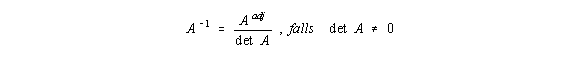The following applies to 3x3 matrices: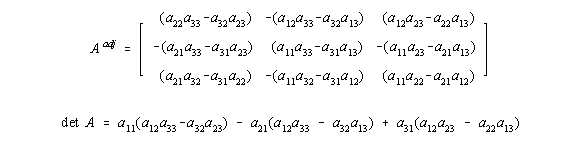### 4) General projections

The coefficients a31 and a32 determine any projections. For a31 or a32 unequal to zero, the mappings are no longer affine, parallelism need not be preserved.

In general, from the equation X '= A * X Obtain the following formulas for the calculation of the image coordinates in two-dimensional: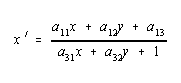The denominator is omitted for affine transformations, since a31 and a32 then are always zero.

Images of this kind are determined by four points with their images. (However, the following applies: not all images that are given by four points with their images can be represented in homogeneous coordinates with a 3x3 matrix!)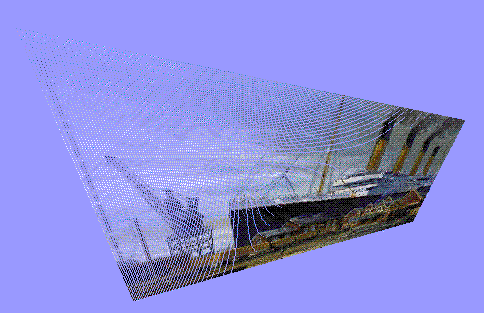Illustration with the matrix:Parallels remain Not received, this is not an affine mapping. (The holes in the picture are only from the forward figure.)

### 5) interpolation

Interpolation is always necessary when the rasterization of the original image and image do not match, i.e. the computational target of image points does not exactly match the rasterization in the image, or pixels are not hit or are hit several times. Ideally, it should be possible to calculate the original image back from the image. This is not possible. if information is falsified or lost due to unsuitable interpolation methods. If the number of pixels is reduced, a re-enlargement cannot of course result in the original image. When enlarged, the additional pixels have to be filled in, which leads to a staircase effect. The recalculation of the original will only ever be possible approximately. It is therefore necessary to consider beforehand which interpolation method corresponds most closely to the desired requirements. Based on the discrete pixel structure of an image, a suitable continuous function is first designed that comes as close as possible to this gray value distribution, e.g. a polynomial through the given points of the image. This convolution function must therefore calculate gray values ​​based on the neighborhood relationships.

Let x be a point and xm, n one from the neighborhood of x. 'm', 'n' relate to the position within the filter kernel, applied to x. G (xm, n) is the gray value of xm, n:H is the filter core used, Gi(x) the resulting gray value at point i.

If we assume that the filter core H is separable, it can be broken down into one-dimensional filter cores.

### 5.1) Interpolation with polynomials

Polynomials of the nth degree are put through the given points. The polynomials represent the approximation.

A higher degree gives a smoother curve, but also requires more computing time. The simplest case is n = 0. No mean values ​​at all are calculated here, but the values ​​of the interpolation points are used. The "compensating" curve consists of constants and has jumps, so it is not continuous. These jumps can be seen in the image as gray value levels.

In case n = 1, all points are connected with straight lines, where x = 0 is the point to be interpolated.

Let dx be the distance between two neighboring points, then for -dx / 2 :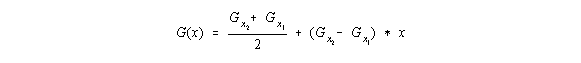The following results for the filter core: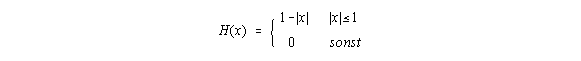The compensating curve has no jumps but corners at the support points, so it is continuous but not differentiable. The picture is much softer compared to the previous case.

From n = 2, parabolas are used.No interpolation takes place for n = 0, the points receive the value of the nearest neighbor.

There are no jumps for n = 1, but the curve in the interpolation points cannot be continuously derived.

For n> 1, a curved curve then results with increased computational effort.

The disadvantage of interpolating with polynomials is that not all derivatives are continuous.Enlargement by a factor of 4 using the closest neighbors.

Every four pixels in a line receive the same value, which creates a staircase effect.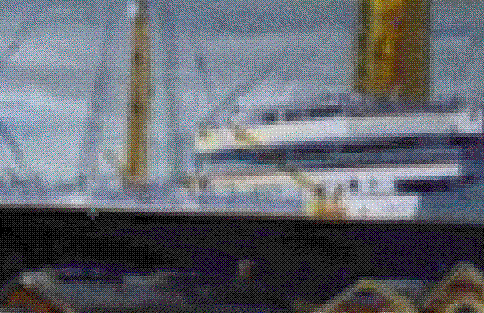Enlargement by a factor of 4. The pixel values ​​are calculated from the original image pixels with their four neighbors. A type of linear interpolation is used, the arithmetic mean of the intensities of the three color components. The staircase effect is significantly smoothed out. (The distances to the neighbors are not included in this example.)

### 5.2) Interpolation with B-splines

With B-splines it is possible to produce an interpolation that is continuous in all derivatives, which may be an advantage.

A B-spline is a compensating curve around predetermined points P.i and is calculated as a parameterized curve in principle using the following formula:where t from [0,1] and Bi(t) is a weight that can be calculated recursively.

Thiefi(t) belong to a previously defined order which determines the quality of the interpolation. With order 1, the values ​​of the neighboring pixels are adopted. Order 2 is a linear interpolation, i.e. the points are connected to one another. From order 3, compensating curves result. In the case of B-spline interpolated images, outlier gray values ​​are smoothed, since the curve does not have to run through the interpolation points.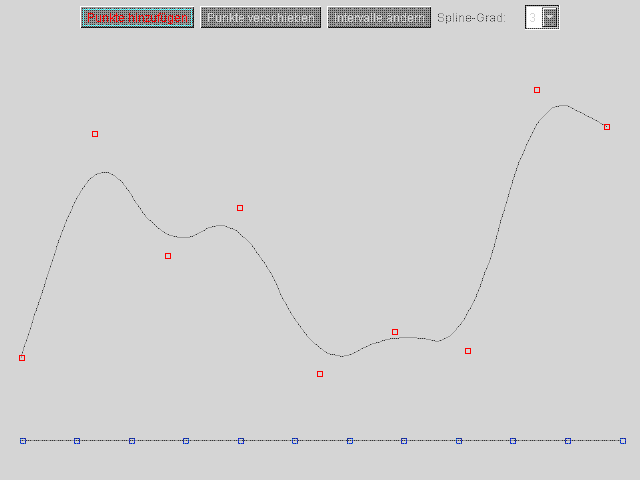B-spline of order 4.

An applet that generates B-splines can be found at:

www.inf.fu-berlin.de/~vratisla/Bildverarbeitung/Bspline/Bspline.html.

### 6.1) scaling

In the event of a reduction, all very fine details that should no longer be visible in the image should be smoothed out beforehand (e.g. with B-splines), otherwise they may be adopted (if the reverse image hits them). Since the pixels in the original image usually do not match those in the image, interpolation must be carried out.

When using an interpolation filter with separable filter cores, i.e. filter cores that can be broken down into one-dimensional cores, all rows can be scaled first and only then the columns, which increases efficiency. For this purpose, the same spatial coefficients can be used for each line, which saves a considerable amount of computing time.

The columns are scaled accordingly in the next step.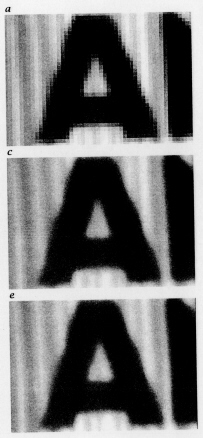Scaled image and a line profile:

a) Enlargement with interpolation of order 0, i.e. with adoption of the neighboring pixels

b) Magnification with interpolation of order 1, i.e. with linear interpolation. (Connecting the pixel values ​​by straight lines)

c) Magnification with interpolation of order 3, i.e. with cubic interpolation (polynomials of order 3)

When the neighboring pixel values ​​are taken over, steps arise. The interpolations of the 1st and 3rd order can hardly be distinguished.

### 6.2) rotation

Here, too, the use of separable filter cores can increase efficiency. Because they make it possible to use one-dimensional operations.

Rotation is possible with a single matrix multiplication, but rows and columns are transformed at the same time. However, it is possible to carry out this operation with a series of one-dimensional transformations in order to be able to couple it with the one-dimensional filter operations. For this one can use three shearings:Shearing can be carried out efficiently line by line, since the same coefficients can be used for each line, as with scaling.

### 6.3) Arbitrary affine mappings

Each linear mapping can be represented with a 2x2 matrix and can be broken down into scaling, shear and rotation.The rotation can be decomposed as described above. The scaling parameters sx and sy, the shear parameter s, and the angle of rotation can be calculated as follows:For an efficient affine transformation, a maximum of three operations are required for the rotation component, one each for scaling and shear and one for translation. Each of these simple two-dimensional operations is coupled with the interpolation filter.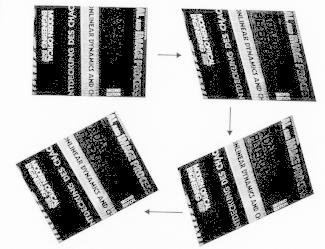Efficient rotation made up of three shears.

### 7) Finally, an applet

Finally, various transformations with the use of the inverse mapping are applied for an applet that allows a view of a flat landscape while imitating the natural curvature of the earth. The applet is under

www.inf.fu-berlin.de/~vratisla/Flug/Flug.html available.

A map is projected onto a spherical surface, which in turn is mapped onto the imaginary screen with central projection.

A sequence of images from the applet www.inf.fu-berlin.de/~vratisla/Flug/Flug.html.

Jähne, chapter 8